Optics

# Refractive index

The refractive index is a dimensionless quantity that measures the ratio between the speeds of light in different media.

Refractive index is the ratio of the speeds of light between two media through which it travels. The absolute refractive index , in turn, is calculated with reference to the vacuum , the medium through which light propagates with the greatest possible speed. Therefore, we can say that this index measures how many times the speed of light is greater in a vacuum than in other media, while the relative refractive index is defined as the ratio between the speeds of light between any two media .

The refractive index is a scalar , dimensionless quantity whose modulus is always greater than or equal to 1 . For example, the speed of light in a vacuum is 1.33 times greater than that of light traveling in a medium whose refractive index is 1.33. Furthermore, there is no knowledge of any medium in which light travels faster than in a vacuum.

Furthermore, the refractive index depends directly on the frequency of light incident on the medium. Generally, the higher the frequency of light , the higher the refractive index of light for that specific frequency. It is for this reason that light is dispersed and begins to display the colors of the visible spectrum, for example, when propagating through a prism.

## How to calculate the refractive index?

The refractive index can be calculated by the following formula, please note: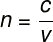Subtitle:

n — refractive index

c — speed of light in vacuum

v — speed of light in the medium

In the equation just presented, c is the speed of light in vacuum, whose magnitude is approximately 299,792,458 m/s (3.0 x 10 8 m/s), about three hundred million meters per second. The variable v is the speed of light in the other media in which it propagates, such as in air, water or glass. We say that a medium that has a higher refractive index than the refractive index of other media is more refractive.

Examples

Check out some solved examples that show us how it is possible to make calculations related to the refractive index:

1) Let’s calculate the refractive index of light that propagates inside a diamond. To do so, consider that, inside the diamond, light propagates with a speed of 1.25 x 10 8 m/s, see how: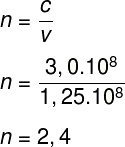The result indicates that, in a vacuum, light travels 2.4 times faster than inside a diamond.

2) A certain transparent and homogeneous medium has an absolute refractive index of 1.2. Determine the speed at which light travels through it.

Data : c = 3.0.10 8 m/s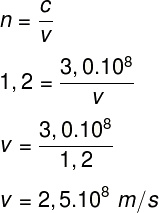## Refractive index table

Check out a table that presents the refractive indices of some known materials:

 Material Refractive index Air 1.0003 Water 1.33 Ice 1.31 Alcohol 1.36 Oil 1.46 crown glass 1.52 Diamond 2.42 Ruby 1.71

## Absolute and relative refractive index

We call the absolute refractive index the ratio between the speeds of light in a vacuum and in a given medium. The relative refractive index , in turn, is defined as the ratio between the speed of light that propagates between two media, provided that one of them is not a vacuum.

We can calculate the relative refractive index using the following equation: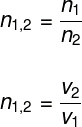In this equation, the medium, whose index of refraction is given by n 1 , is the medium from which the light comes, while the medium of refractive index n 2 is the medium towards which the light is directed.

## Exercises solved

1) In a certain transparent material, light propagates with a speed equal to 0.8c, being c the speed of light in vacuum. Determine the refractive index of this material.

Resolution:

To solve this exercise, we will use the refractive index formula: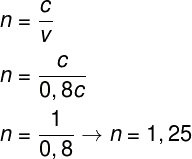2) In a certain material, light travels at 75% of the speed of light. Determine the refractive index of this material.

Resolution:

To solve the exercise, we need to consider that 75% of the speed of light can be written as 0.75c, where c represents the speed of light in vacuum. Next, we will use the refractive index formula: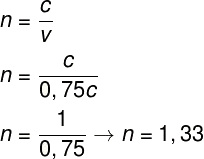3) Light propagates between two media of different refractive indices. On its way, light emerges from a medium whose index of refraction is 1.5 towards a medium whose index of refraction is 1.25. Determine the relative refractive index for these two media.

Resolution:

Using the refractive indices informed in the statement, we will calculate the relative refractive index according to the following calculation: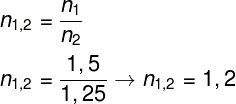The result obtained — 1.2 — indicates that the speed of light is 20% greater in medium 2 than in medium 1.